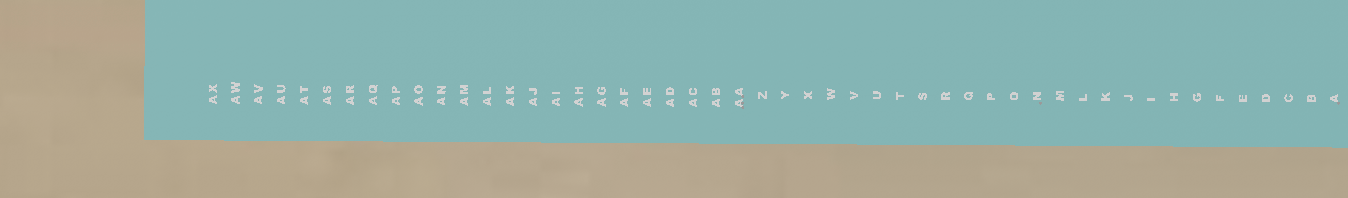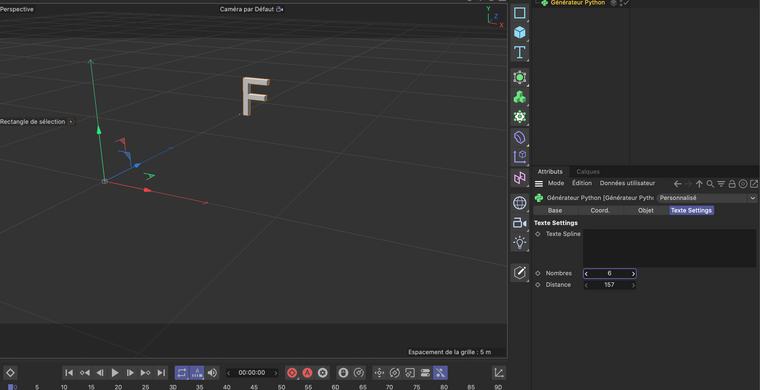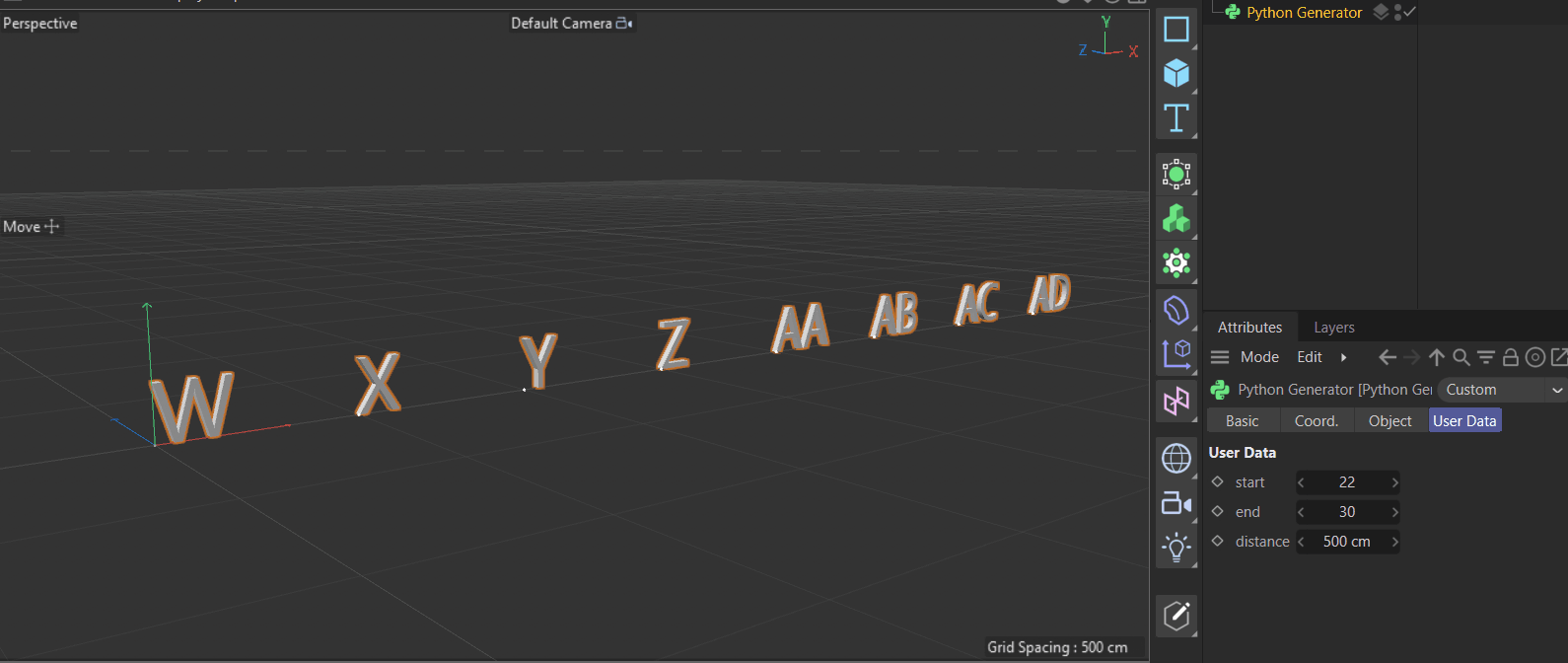# Solved Python Generator and texte

Hello there,

for the plugin i'm working on, I need to generate an aeronautical numerotations. Meaning it goes like A, B , C... Z, AA, AB , ACI made a code wich I fill with the number of letters I want and the distance I want between each letters.

``````import c4d

def main():

number= op [c4d.ID_USERDATA,3]
distance = op [c4d.ID_USERDATA,4]
alphabet=["A","B","C","D","E","F","G","H","I","J","K","L","M","N","O","P","Q","R","S","T","U","V","W","X","Y","Z"] ;

for x in range( number):

if ((x//26)-1==-1) :
null = c4d.BaseObject ( c4d.Onull)
texte = c4d.BaseObject(1019268)
texte[c4d.PRIM_TEXT_TEXT]= alphabet [x]
print (alphabet [x])
texte.InsertUnder ( null)
else :
null = c4d.BaseObject ( c4d.Onull)
texte = c4d.BaseObject(1019268)
texte[c4d.PRIM_TEXT_TEXT]= alphabet [(x//26 - 1)]; alphabet [x%26]
print (alphabet [x//26 - 1], alphabet [x%26])
texte.InsertUnder ( null)
texte[c4d.ID_BASEOBJECT_REL_POSITION,c4d.VECTOR_Z] = x* distance

return null
``````

This is the result of my programs. I have the right letters but it display only the letters corrrespodings to the number not all letter before. Like in the picture above.I hope my issue is clear

Thanks a lot

Hello @bureaudesprojets,

welcome to the Plugin Café and thank you for reaching out to us. That looks like a fun project you have there.

However, there are a few problems with your code.

You redefine your `null` on each step of the loop, causing only the last iteration to be returned. It is:

``````if ((x // 26)-1 == -1):
null = c4d.BaseObject(c4d.Onull)
...
texte.InsertUnder(null)
else:
null = c4d.BaseObject(c4d.Onull)
...
texte.InsertUnder(null)
``````

But it should be:

``````null = c4d.BaseObject(c4d.Onull)
for x in range( number):
if ((x // 26)-1 == -1):
...
texte.InsertUnder(null)
else:
...
texte.InsertUnder(null)
``````

This was what caused your major problem, but there were also other problems with how you set the distance between the elements with `texte[c4d.ID_BASEOBJECT_REL_POSITION,c4d.VECTOR_Z] = x* distance`, which was outside of the loop, and I also did not understand the whole "aeronautical numerations" thing in your code.

Find below an example of how I would do it.

Cheers,
Ferdinand

The result:The file:
abcd.c4d

The code:

``````"""Simple example for building caches for a Python Generator object.
"""

import c4d
import itertools

# The upper case letters from their ASCII range.
ALPHABET = tuple(chr (i) for i in range(65, 91))

def GetNumerationList(a: int, b: int) -> list[str]:
"""Returns a list of numerations following your scheme from index #a
to #b.
"""
# I have no clue what aeronautical numerations are, but I guess
# you just want A-Z, AA-AZ, BA-BZ, ..., ZA-ZZ, AAA - AAZ, and so on.
# I do not really see how your code was supposed to do that. I did
# provide below a simple version using itertools.

assert(a < b)

# Build the full array, we could also be more elegant here, and only
# build what we need, but that would be up to you. I create here quite
# a lot of entries to avoid index errors. range(1, 4) means we create
# everything from A to ZZZ, i.e., 26 + 26² + 26³ ~ 18,000 items. Python's
# iteration is slow, and you should optimize this.
result = []
for i in range(1, 4):
result += ("".join(item)
for item in itertools.product(ALPHABET, repeat=i))

# And return the slice we have been asked for.
return result[a:b]

def main():
"""Executed by Cinema 4D to build the cache of the Python Generator.
"""
# Instantiate a null object to parent the "numerations" to.
null = c4d.BaseObject(c4d.Onull)
if not isinstance(null, c4d.BaseObject):
raise MemoryError()

# Get the user data.
a = op[c4d.ID_USERDATA, 1] # The starting index.
b = op[c4d.ID_USERDATA, 2] # The end index.
distance = op[c4d.ID_USERDATA, 3] # The distance between elements.

# Iterate over all numerations between the index #a and #b.
for i, item in enumerate(GetNumerationList(a, b)):
# Instantiate a text object.
node = c4d.BaseObject(1019268)
if not isinstance(node, c4d.BaseObject):
raise MemoryError("Could not allocate object.")

# Set the text to the yielded numeration item.
node[c4d.PRIM_TEXT_TEXT] = item
# Set the distance on the x-axis in the local space of the parent,
# the null object.
node.SetMl(c4d.Matrix(off=c4d.Vector(i * distance, 0, 0)))
# Parent the text object to the null object.
node.InsertUnder(null)

# Return the cache.
return null
``````

MAXON SDK Specialist
developers.maxon.net

Hello,

Thanks a lot for your help !